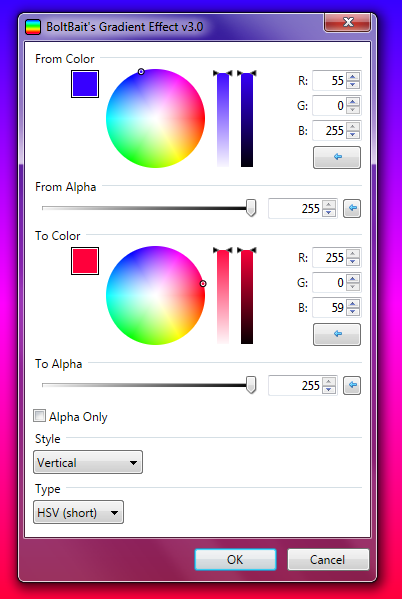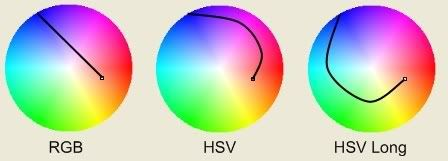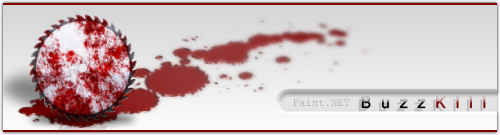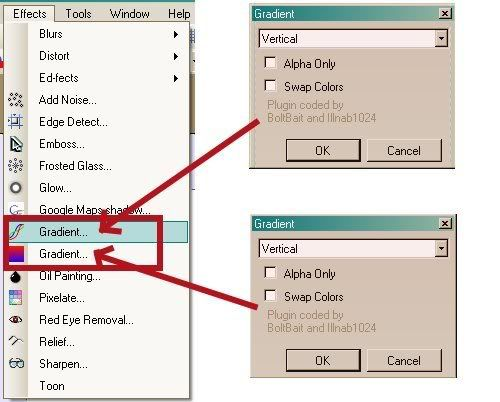# BoltBait & Illnab1024 Gradient Plugin (UPDATED!) v3.0

## Recommended PostsPaint.NET 3.x users look here:

Hidden Content:

Download (v2.0) DLL here: Gradient.dll  MODERATORS NOTE:  This effect is incompatible with Paint.NET 4.0x[/size]

Okay. There is already Pleska's plugin, but that one is slightly buggy. This Plugin (From Scratch), works out a few kinks in Pleska's plugin, and includes a few more gradient types.

Version 2.0 brought in Hue-based gradients. These create beautiful rainbow gradients that are based on a color's hue, saturation, and value.

Here are the gradient types:Here are all of the gradient styles:

VerticalHorizontalDiagonal (Upper Left to Bottom Right)Diagonal (Upper Right to Lower LeftSquareConicalThe Main Render function was coded by BoltBait

The User Interface and Effects implementation was coded by Illnab1024

Hosting provided by Paint.NET

Thanks to EvanOlds for letting us use his HSVtoRGB, RGBtoHSV, and AngleConstrain functions. They were needed (rather than using the Paint.NET functions; these broke it down better).

In addition, gratitude should be served to pleska for providing the first Gradient function, and Crazy Man Dan for the first Gradient Tutorial.

Here is the CodeLab source for the 4.0 version (avert your eyes!):

Hidden Content:

```// Title: BoltBait's Gradient Effect v3.0
// Author: BoltBait
// Desc: Render various colorful gradients
// URL: http://www.BoltBait.com/pdn
#region UICode
ColorBgra Amount1 = ColorBgra.FromBgr(0,0,0); // From Color
int Amount2 = 255; // [0,255] From Alpha
ColorBgra Amount3 = ColorBgra.FromBgr(255,255,255); // To Color
int Amount4 = 255; // [0,255] To Alpha
bool Amount5 = false; // [0,1] Alpha Only
byte Amount6 = 0; // Style|Vertical|Horizontal|Diagonal|Diagnal (other)|Radial Corner|Radial Side|Square|Conical
byte Amount7 = 0; // Type|RGB|HSV (short)|HSV (long)
#endregion

// Here is the main render loop function
void Render(Surface dst, Surface src, Rectangle rect)
{
bool AlphaOnly = Amount5; // false=full color, true=alpha channel only
int Direction = Amount6;      // 0=vertical, 1=horizontal, 2-3=diagonal, 4=Radial Corner, 5=Radial Side, 6=square, 7=conical
bool SwapColors = false;     // 0=normal, 1=swapped

int FadeType = Amount7;

ColorBgra PrimaryColor, SecondaryColor, currpixel;
double xD, yD;
double ceiling, outer;
int FirstR, FirstG, FirstB, FirstAlpha;
int MaxR, MaxG, MaxB, MaxAlpha;
double MidR, MidG, MidB, MidAlpha;
int RowR, RowG, RowB, RowAlpha;
double MaxDistance = 0, CurrDistance = 0;
long CenterX = 0, CenterY = 0;
double angle = 0, XPercent = 0, YPercent = 0;
double FirstH = 0, FirstS = 0, FirstV = 0;
double LastH = 0, LastS = 0, LastV = 0;
double MaxH = 0, MaxS = 0, MaxV = 0;
int HAdjustmentDirection = 1, SAdjustmentDirection = 1, VAdjustmentDirection = 1;
double MidH = 0, MidS = 0, MidV = 0;
double RowH = 0, RowS = 0, RowV = 0;
Rectangle selection = EnvironmentParameters.GetSelection(src.Bounds).GetBoundsInt();

PrimaryColor = Amount1;
PrimaryColor.A = (byte)Amount2;
SecondaryColor = Amount3;
SecondaryColor.A = (byte)Amount4;

FirstAlpha = PrimaryColor.A;
MaxAlpha = Math.Abs(SecondaryColor.A - PrimaryColor.A);

if (PrimaryColor.A > SecondaryColor.A)
{
}

if (((PrimaryColor.R == PrimaryColor.G) && (PrimaryColor.R == PrimaryColor.) ||
((SecondaryColor.R == SecondaryColor.G) && (SecondaryColor.R == SecondaryColor.))
{
}

FirstR = PrimaryColor.R;
FirstG = PrimaryColor.G;
FirstB = PrimaryColor.B;

EvanRGBtoHSV(PrimaryColor.R, PrimaryColor.G, PrimaryColor.B, ref FirstH, ref FirstS, ref FirstV);
EvanRGBtoHSV(SecondaryColor.R, SecondaryColor.G, SecondaryColor.B, ref LastH, ref LastS, ref LastV);

MaxR = Math.Abs(SecondaryColor.R - PrimaryColor.R);
MaxG = Math.Abs(SecondaryColor.G - PrimaryColor.G);
MaxB = Math.Abs(SecondaryColor.B - PrimaryColor.;

if (PrimaryColor.R > SecondaryColor.R)
{
}
if (PrimaryColor.G > SecondaryColor.G)
{
}
if (PrimaryColor.B > SecondaryColor.
{
}

{
case 1: // Calculate MaxH and adjustment direction for short HSV fades
if (Math.Abs(FirstH - LastH) > 180.0)
{ // short will be wrapping around 0
if (FirstH > LastH)
{
MaxH = 360.0 - FirstH + LastH;
}
else
{
MaxH = 360.0 - LastH + FirstH;
}
}
else
{ // short will NOT be wrapping
MaxH = Math.Abs(FirstH - LastH);
if (FirstH > LastH)
{
}
}
break;
case 2: // Calculate MaxH and adjustment direction for long HSV fades
if (Math.Abs(FirstH - LastH) > 180.0)
{ // long will NOT be wrapping
MaxH = Math.Abs(FirstH - LastH);
if (FirstH > LastH)
{
}
}
else
{ // long will be wrapping
if (FirstH > LastH)
{
MaxH = 360.0 - FirstH + LastH;
}
else
{
MaxH = 360.0 - LastH + FirstH;
}
}
break;
default:
break;
}
MaxS = Math.Abs(FirstS - LastS);
if (FirstS > LastS) SAdjustmentDirection = -1;
MaxV = Math.Abs(FirstV - LastV);
if (FirstV > LastV) VAdjustmentDirection = -1;

MidR = (FirstR + (RAdjustmentDirection * (MaxR / 2)));
MidG = (FirstG + (GAdjustmentDirection * (MaxG / 2)));
MidB = (FirstB + (BAdjustmentDirection * (MaxB / 2)));
MidAlpha = (FirstAlpha + (AlphaAdjustmentDirection * (MaxAlpha / 2)));

MidH = AngleConstrain(FirstH + (HAdjustmentDirection * (MaxH / 2)));
MidS = (FirstS + (SAdjustmentDirection * (MaxS / 2)));
MidV = (FirstV + (VAdjustmentDirection * (MaxV / 2)));

ceiling = selection.Bottom - selection.Top;
outer = selection.Right - selection.Left;
CenterX = (long)(((selection.Right - selection.Left) / 2) + selection.Left);
CenterY = (long)(((selection.Bottom - selection.Top) / 2) + selection.Top);

switch (Direction)
{
case 4: //corner
MaxDistance = (double)Math.Sqrt((CenterX - selection.Left) * (CenterX - selection.Left) + (CenterY - selection.Top) * (CenterY - selection.Top));
break;
case 5: //short side
MaxDistance = (double)Math.Min(CenterX - selection.Left, CenterY - selection.Top);
break;
default:
break;
}

for (int y = rect.Top; y < rect.Bottom; y++)
{
if (IsCancelRequested) return;
for (int x = rect.Left; x < rect.Right; x++)
{
currpixel = src[x,y];

xD = (int)x;
yD = (int)y;
switch (Direction)
{
case 1:
if (!AlphaOnly)
{
{
case 0:
currpixel.R = (byte)(FirstR + (RAdjustmentDirection * ((xD - selection.Left) / outer * MaxR)));
currpixel.G = (byte)(FirstG + (GAdjustmentDirection * ((xD - selection.Left) / outer * MaxG)));
currpixel.B = (byte)(FirstB + (BAdjustmentDirection * ((xD - selection.Left) / outer * MaxB)));
break;
case 1:
case 2:
EvanHSVtoRGB(AngleConstrain(FirstH + (HAdjustmentDirection * ((xD - selection.Left) / outer * MaxH))),
FirstS + (SAdjustmentDirection * ((xD - selection.Left) / outer * MaxS)),
FirstV + (VAdjustmentDirection * ((xD - selection.Left) / outer * MaxV)),
ref currpixel.R, ref currpixel.G, ref currpixel.;
break;
}
}
currpixel.A = (byte)(FirstAlpha + (AlphaAdjustmentDirection * ((xD - selection.Left) / outer * MaxAlpha)));
break;
case 0:
if (!AlphaOnly)
{
{
case 0:
currpixel.R = (byte)(FirstR + (RAdjustmentDirection * ((yD - selection.Top) / ceiling * MaxR)));
currpixel.G = (byte)(FirstG + (GAdjustmentDirection * ((yD - selection.Top) / ceiling * MaxG)));
currpixel.B = (byte)(FirstB + (BAdjustmentDirection * ((yD - selection.Top) / ceiling * MaxB)));
break;
case 1:
case 2:
EvanHSVtoRGB(AngleConstrain(FirstH + (HAdjustmentDirection * ((yD - selection.Top) / ceiling * MaxH))),
FirstS + (SAdjustmentDirection * ((yD - selection.Top) / ceiling * MaxS)),
FirstV + (VAdjustmentDirection * ((yD - selection.Top) / ceiling * MaxV)),
ref currpixel.R, ref currpixel.G, ref currpixel.;
break;
}
}
currpixel.A = (byte)(FirstAlpha + (AlphaAdjustmentDirection * ((yD - selection.Top) / ceiling * MaxAlpha)));
break;
case 2:
if (!AlphaOnly)
{
{
case 0:
RowR = (byte)(FirstR + (RAdjustmentDirection * ((yD - selection.Top) / ceiling * (MaxR / 2))));
RowG = (byte)(FirstG + (GAdjustmentDirection * ((yD - selection.Top) / ceiling * (MaxG / 2))));
RowB = (byte)(FirstB + (BAdjustmentDirection * ((yD - selection.Top) / ceiling * (MaxB / 2))));
currpixel.R = (byte)(RowR + (RAdjustmentDirection * ((xD - selection.Left) / outer * (MaxR / 2))));
currpixel.G = (byte)(RowG + (GAdjustmentDirection * ((xD - selection.Left) / outer * (MaxG / 2))));
currpixel.B = (byte)(RowB + (BAdjustmentDirection * ((xD - selection.Left) / outer * (MaxB / 2))));
break;
case 1:
case 2:
RowH = (FirstH + (HAdjustmentDirection * ((yD - selection.Top) / ceiling * (MaxH / 2))));
RowS = (FirstS + (SAdjustmentDirection * ((yD - selection.Top) / ceiling * (MaxS / 2))));
RowV = (FirstV + (VAdjustmentDirection * ((yD - selection.Top) / ceiling * (MaxV / 2))));
EvanHSVtoRGB(AngleConstrain(RowH + (HAdjustmentDirection * ((xD - selection.Left) / outer * (MaxH / 2)))),
RowS + (SAdjustmentDirection * ((xD - selection.Left) / outer * (MaxS / 2))),
RowV + (VAdjustmentDirection * ((xD - selection.Left) / outer * (MaxV / 2))),
ref currpixel.R, ref currpixel.G, ref currpixel.;
break;
}
}
RowAlpha = (byte)(FirstAlpha + (AlphaAdjustmentDirection * ((yD - selection.Top) / ceiling * (MaxAlpha / 2))));
currpixel.A = (byte)(RowAlpha + (AlphaAdjustmentDirection * ((xD - selection.Left) / outer * (MaxAlpha / 2))));
break;
case 3:
if (!AlphaOnly)
{
{
case 0:
RowR = (byte)(MidR + (RAdjustmentDirection * ((yD - selection.Top) / ceiling * (MaxR / 2))));
RowG = (byte)(MidG + (GAdjustmentDirection * ((yD - selection.Top) / ceiling * (MaxG / 2))));
RowB = (byte)(MidB + (BAdjustmentDirection * ((yD - selection.Top) / ceiling * (MaxB / 2))));
currpixel.R = (byte)(RowR - (RAdjustmentDirection * ((xD - selection.Left) / outer * (MaxR / 2))));
currpixel.G = (byte)(RowG - (GAdjustmentDirection * ((xD - selection.Left) / outer * (MaxG / 2))));
currpixel.B = (byte)(RowB - (BAdjustmentDirection * ((xD - selection.Left) / outer * (MaxB / 2))));
break;
case 1:
case 2:
RowH = (MidH + (HAdjustmentDirection * ((yD - selection.Top) / ceiling * (MaxH / 2))));
RowS = (MidS + (SAdjustmentDirection * ((yD - selection.Top) / ceiling * (MaxS / 2))));
RowV = (MidV + (VAdjustmentDirection * ((yD - selection.Top) / ceiling * (MaxV / 2))));
EvanHSVtoRGB(AngleConstrain(RowH - (HAdjustmentDirection * ((xD - selection.Left) / outer * (MaxH / 2)))),
RowS - (SAdjustmentDirection * ((xD - selection.Left) / outer * (MaxS / 2))),
RowV - (VAdjustmentDirection * ((xD - selection.Left) / outer * (MaxV / 2))),
ref currpixel.R, ref currpixel.G, ref currpixel.;
break;
}
}
RowAlpha = (byte)(MidAlpha + (AlphaAdjustmentDirection * ((yD - selection.Top) / ceiling * (MaxAlpha / 2))));
currpixel.A = (byte)(RowAlpha - (AlphaAdjustmentDirection * ((xD - selection.Left) / outer * (MaxAlpha / 2))));
break;
case 4:
case 5:
CurrDistance = (double)Math.Sqrt(((CenterX - x) * (CenterX - x)) + ((CenterY - y) * (CenterY - y)));
if (CurrDistance < MaxDistance)
{
if (!AlphaOnly)
{
{
case 0:
currpixel.R = (byte)(FirstR + (RAdjustmentDirection * (CurrDistance / MaxDistance * MaxR)));
currpixel.G = (byte)(FirstG + (GAdjustmentDirection * (CurrDistance / MaxDistance * MaxG)));
currpixel.B = (byte)(FirstB + (BAdjustmentDirection * (CurrDistance / MaxDistance * MaxB)));
break;
case 1:
case 2:
EvanHSVtoRGB(AngleConstrain(FirstH + (HAdjustmentDirection * (CurrDistance / MaxDistance * MaxH))),
FirstS + (SAdjustmentDirection * (CurrDistance / MaxDistance * MaxS)),
FirstV + (VAdjustmentDirection * (CurrDistance / MaxDistance * MaxV)),
ref currpixel.R, ref currpixel.G, ref currpixel.;
break;
}
}
currpixel.A = (byte)(FirstAlpha + (AlphaAdjustmentDirection * (CurrDistance / MaxDistance * MaxAlpha)));
}
else
{
if (!AlphaOnly)
{
currpixel.R = SecondaryColor.R;
currpixel.G = SecondaryColor.G;
currpixel.B = SecondaryColor.B;
}
currpixel.A = SecondaryColor.A;
}
break;
case 6:
XPercent = Math.Min(Math.Abs(xD - selection.Left), Math.Abs(xD - selection.Right)) / (CenterX - selection.Left);
YPercent = Math.Min(Math.Abs(yD - selection.Top), Math.Abs(yD - selection.Bottom)) / (CenterY - selection.Top);
if (XPercent > YPercent)
{
if (!AlphaOnly)
{
{
case 0:
currpixel.R = (byte)(FirstR + (RAdjustmentDirection * YPercent * MaxR));
currpixel.G = (byte)(FirstG + (GAdjustmentDirection * YPercent * MaxG));
currpixel.B = (byte)(FirstB + (BAdjustmentDirection * YPercent * MaxB));
break;
case 1:
case 2:
EvanHSVtoRGB(AngleConstrain(FirstH + (HAdjustmentDirection * YPercent * MaxH)),
FirstS + (SAdjustmentDirection * YPercent * MaxS),
FirstV + (VAdjustmentDirection * YPercent * MaxV),
ref currpixel.R, ref currpixel.G, ref currpixel.;
break;
}
}
currpixel.A = (byte)(FirstAlpha + (AlphaAdjustmentDirection * YPercent * MaxAlpha));
}
else
{
if (!AlphaOnly)
{
{
case 0:
currpixel.R = (byte)(FirstR + (RAdjustmentDirection * XPercent * MaxR));
currpixel.G = (byte)(FirstG + (GAdjustmentDirection * XPercent * MaxG));
currpixel.B = (byte)(FirstB + (BAdjustmentDirection * XPercent * MaxB));
break;
case 1:
case 2:
EvanHSVtoRGB(AngleConstrain(FirstH + (HAdjustmentDirection * XPercent * MaxH)),
FirstS + (SAdjustmentDirection * XPercent * MaxS),
FirstV + (VAdjustmentDirection * XPercent * MaxV),
ref currpixel.R, ref currpixel.G, ref currpixel.;
break;
}
}
currpixel.A = (byte)(FirstAlpha + (AlphaAdjustmentDirection * XPercent * MaxAlpha));
}
break;
case 7:
xD = xD - CenterX;
yD = yD - CenterY;
angle = Math.Atan2(yD, xD) * (180 / Math.PI);
if (angle < 0) angle *= -1; // should make a mirror image on the bottom
if (!AlphaOnly)
{
{
case 0:
currpixel.R = (byte)(FirstR + (RAdjustmentDirection * (angle / 180 * MaxR)));
currpixel.G = (byte)(FirstG + (GAdjustmentDirection * (angle / 180 * MaxG)));
currpixel.B = (byte)(FirstB + (BAdjustmentDirection * (angle / 180 * MaxB)));
break;
case 1:
case 2:
EvanHSVtoRGB(AngleConstrain(FirstH + (HAdjustmentDirection * (angle / 180 * MaxH))),
FirstS + (SAdjustmentDirection * (angle / 180 * MaxS)),
FirstV + (VAdjustmentDirection * (angle / 180 * MaxV)),
ref currpixel.R, ref currpixel.G, ref currpixel.;
break;
}
}
currpixel.A = (byte)(FirstAlpha + (AlphaAdjustmentDirection * (angle / 180 * MaxAlpha)));
break;
default:
break;
}

dst[x,y] = currpixel;
}
}
}

public void EvanHSVtoRGB(double H, double S, double V, ref byte bR, ref byte bG, ref byte bB)
{
const double HSV_UNDEFINED = -999.0;
// Parameters must satisfy the following ranges:
// 0.0 <= H < 360.0
// 0.0 <= S <= 1.0
// 0.0 <= V <= 1.0

// Handle special case first
if (S == 0.0 || H == HSV_UNDEFINED)
{
byte x = (byte)(int)(V * 255.0);
bR = x;
bG = x;
bB = x;
return;
}

if (H >= 360.0)
{
H = AngleConstrain(H);
}

double R = V, G = V, B = V;
double Hi = Math.Floor(H / 60.0);
double f = H / 60.0 - Hi;
double p = V * (1.0 - S);
double q = V * (1.0 - f * S);
double t = V * (1.0 - (1.0 - f) * S);
if (Hi == 0.0)
{
R = V;
G = t;
B = p;
}
else if (Hi == 1.0)
{
R = q;
G = V;
B = p;
}
else if (Hi == 2.0)
{
R = p;
G = V;
B = t;
}
else if (Hi == 3.0)
{
R = p;
G = q;
B = V;
}
else if (Hi == 4.0)
{
R = t;
G = p;
B = V;
}
else if (Hi == 5.0)
{
R = V;
G = p;
B = q;
}

int iR = (int)(R * 255.0);
int iG = (int)(G * 255.0);
int iB = (int)(B * 255.0);
bR = (byte)iR;
bG = (byte)iG;
bB = (byte)iB;
}

public void EvanRGBtoHSV(int R, int G, int B, ref double outH, ref double outS, ref double outV)
{
const double HSV_UNDEFINED = -999.0;
// R, G, and B must range from 0 to 255
// Ouput value ranges:
//  outH - 0.0 to 360.0
//  outS - 0.0 to 1.0
//  outV - 0.0 to 1.0

double dR = (double)R / 255.0;
double dG = (double)G / 255.0;
double dB = (double)B / 255.0;
double dmaxRGB = EvanMax3(dR, dG, dB);
double dminRGB = EvanMin3(dR, dG, dB);
double delta = dmaxRGB - dminRGB;

// Set value
outV = dmaxRGB;

// Handle special case
if (dmaxRGB == 0)
{
outH = HSV_UNDEFINED;
outS = 0.0;
return;
}

outS = delta / dmaxRGB;
if (dmaxRGB == dminRGB)
{
outH = HSV_UNDEFINED;
return;
}

// Finally, compute hue
if (dR == dmaxRGB)
{
outH = (dG - dB) / delta * 60.0;
}
else if (dG == dmaxRGB)
{
outH = (2.0 + (dB - dR) / delta) * 60.0;
}
else //if (dB == dmaxRGB)
{
outH = (4.0 + (dR - dG) / delta) * 60.0;
}

if (outH < 0)
{
outH += 360.0;
}
}

public double EvanMax3(double x, double y, double z)
{
return (x > y) ? ((x > z) ? x : z) : ((y > z) ? y : z);
}

public double EvanMin3(double x, double y, double z)
{
return (x < y) ? ((x < z) ? x : z) : ((y < z) ? y : z);
}

public double AngleConstrain(double MyAngle)
{
// Makes sure that 0.0 <= MyAngle < 360.0
// "Wraps around" the value if it's outside this range
if (MyAngle >= 360.0)
{
MyAngle -= Math.Floor(MyAngle / 360.0) * 360.0;
}
if (MyAngle < 0.0)
{
MyAngle += 360.0;
}
return MyAngle;
}
```

~~

##### Share on other sites

My question is if this gradient will work in the space selected, say I want to make a little border, and fade the inner edge darker so it gives it a 3d effect, will it gradient those 2 colors in that space? Or will it gradient the entire image and I will be left with basically one color?

Looks like a great plugin though.##### Share on other sites

Much better than the last one. Square's gonna find some uses.

##### Share on other sites

RaveN: No. I don't even think it is possible with the Current API.

~~

##### Share on other sites

BTW, unlike the other gradiant plugin, this one works with concave selections.

Also, the Radial Side works well with a circle selection.

So does the conical.

@Kaiser Yoshi, yeah, my favorite is square. It really makes a nice sun glint on something if you use a white primary color and a transparent secondary color.

##### Share on other sites

Great work guys!- DO NOT contact me asking for the .pdn of my avatar or the PDN logo. Thank you. Have a nice day.

##### Share on other sites

wo, I had no clue you guys were evan working on something... lmao, anyways, GREAT PLUGINN guys! I love the odd effects...

##### Share on other sites

wo, I had no clue you guys were evan working on something... lmao, anyways, GREAT PLUGINN guys! I love the odd effects...

Haha. Thanks.

Now that I know what I'm doing, more effects on the way.##### Share on other sites

1 question, what one do i add to the effects folder? there are like... 20 icons...

~~

##### Share on other sites

One little problem I have encountered.

I dropped this in effects. It has now overwritten my other gradient plug in.When I choose my old one, this one appears. I really would like to keep both of them.

I liked the bug in the other one, it had some very good uses.##### Share on other sites

Well alright Illnab, I guess I can always just make a new document and paste that in for my borders..I try to refrain from using photoshop as much as I can at home, and I try to use Paint.NET as much as I can. I might have a tutorial coming up with this plugin possibly.##### Share on other sites

T2suggas wrote

I dropped this in effects. It has now overwritten my other gradient plug in.

Same to me .

How can i continue to use the old gradient effect ? I think it was not so bad . :?

To be free To be wild

And to be Just like a child

Mike Oldfield

http://www.myspace.com/tubulartos

##### Share on other sites

it shouldn't overwrite the other one....

Take responsibility for your own intelligence.-Rick Brewster

##### Share on other sites

:? Well i'm going to try to explain with my limited English...when i use the new effect it's ok , the gradient effect appears so i " click" ok and then suddenly the old effect appears too and i can "click " ok or cancel.... Do you understand ? .. :?

To be free To be wild

And to be Just like a child

Mike Oldfield

http://www.myspace.com/tubulartos

##### Share on other sites

Paint.NET 2.xx only distinguishes plugins based on the menu name. 3.0 will be smarter about this.

The Paint.NET Blog: https://blog.getpaint.net/

Donations are always appreciated! https://www.getpaint.net/donate.html

##### Share on other sites

Yeah. I didn't notice this because I have 3.0 and only that (I do have a folder full of 2.72 dll's). I will go ahead and release a new version with a different name...

~~

##### Share on other sites

I will go ahead and release a new version with a different name...

I have tried to rename the plugins like this : Newgradient and Oldgradientthat doesn't workcertainly this is more complicated ...So i'm going to wait for version 3 or an other version if you make one :wink:

I'm already happy to have succeed to use again all the plugins in my Pdn so i can wait for a new plug now :wink:

To be free To be wild

And to be Just like a child

Mike Oldfield

http://www.myspace.com/tubulartos

##### Share on other sites

A new version has been released on the front page with the name change.

~~

##### Share on other sites

Works fine now. Thank you. Great work.--------------

Some people seek justice so persistent, that they will do great injustice themselves.

##### Share on other sites

I have tried to rename the plugins like this : Newgradient and Oldgradientthat doesn't workcertainly this is more complicated ...So i'm going to wait for version 3 or an other version if you make one :wink:

Yeah, Paint.NET 2.xx only distinguishes based on the UI name of the plugin, not the filename. When you click on "Gradient" it goes and finds the first DLL that calls itself "Gradient," regardless of the filename.

3.0 is smarter about thisThe Paint.NET Blog: https://blog.getpaint.net/

Donations are always appreciated! https://www.getpaint.net/donate.html

##### Share on other sites

Version 1.2 released with link, for better distribution.

~~

##### Share on other sitesYeah, Paint.NET 2.xx only distinguishes based on the UI name of the plugin, not the filename. When you click on "Gradient" it goes and finds the first DLL that calls itself "Gradient," regardless of the filename.

this is why you are Rick Brewster and i'm just Tubular TosIn advance , thanks a lot for version 3.0

Out of subjectI have found this link somewhere on the webI think that this video is very funny but I hope this is not disrespectful because i just can understand the word : DevelopersTo be free To be wild

And to be Just like a child

Mike Oldfield

http://www.myspace.com/tubulartos

##### Share on other sites

Thanks for changing it.

I can have my cake and eat it now.## Join the conversation

You can post now and register later. If you have an account, sign in now to post with your account.Reply to this topic...

×   Pasted as rich text.   Paste as plain text instead

Only 75 emoji are allowed.

×   Your previous content has been restored.   Clear editor

×   You cannot paste images directly. Upload or insert images from URL.

×
×